# If z = f(x, y), where x = r + s and y=r – s, show...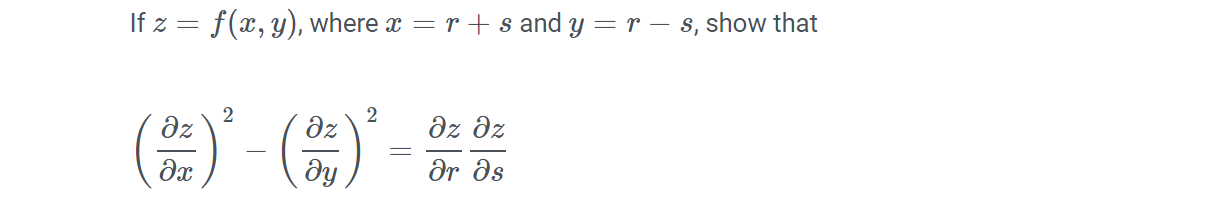If z = f(x, y), where x = r + s and y=r – s, show that 2 2 дz Әх дz ду дz дz მr as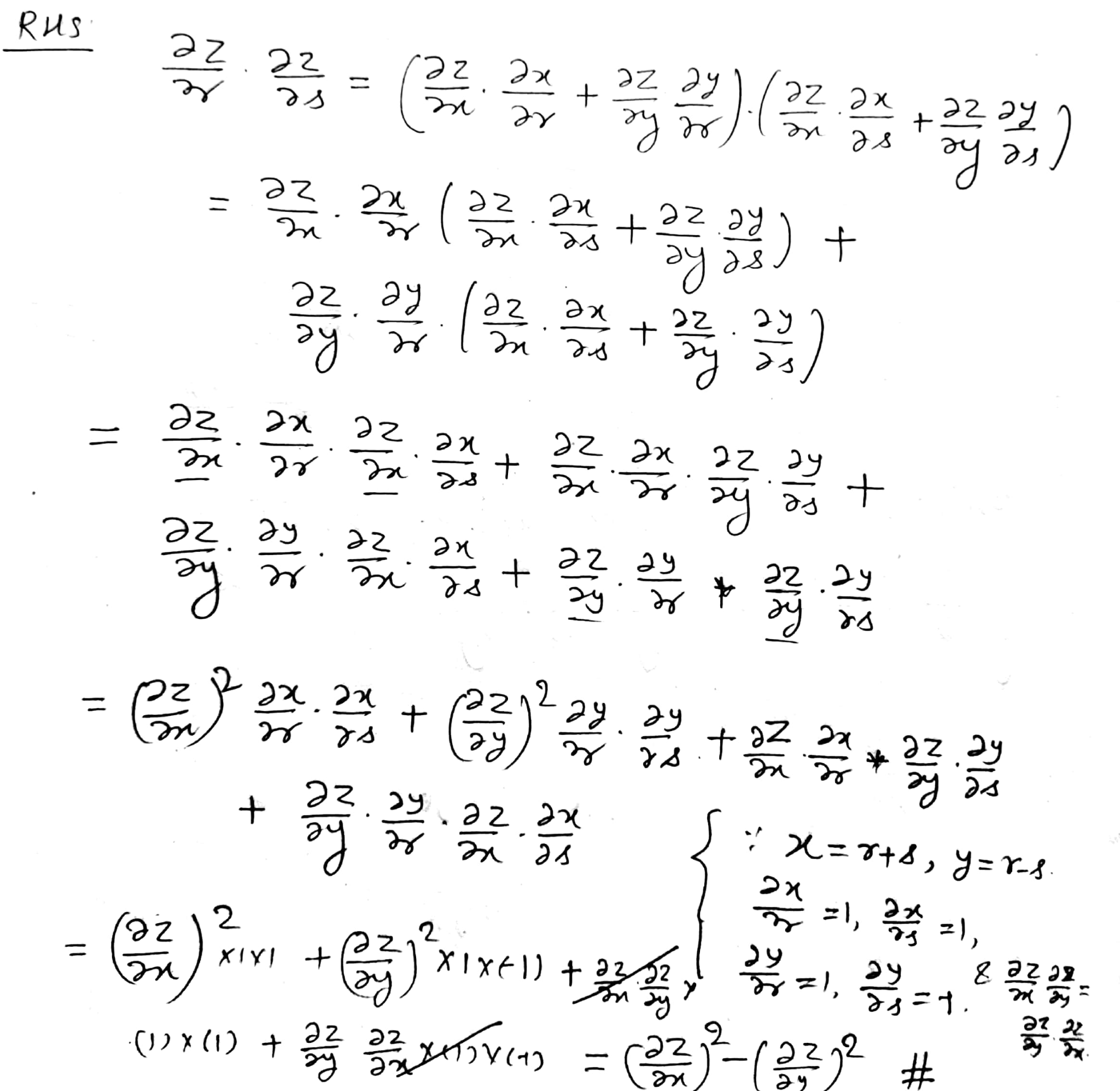#### Earn Coin

Coins can be redeemed for fabulous gifts.

Similar Homework Help Questions
• ### 3. If z = f(x,y), where x = r cos, y=r sin 0 show that 222...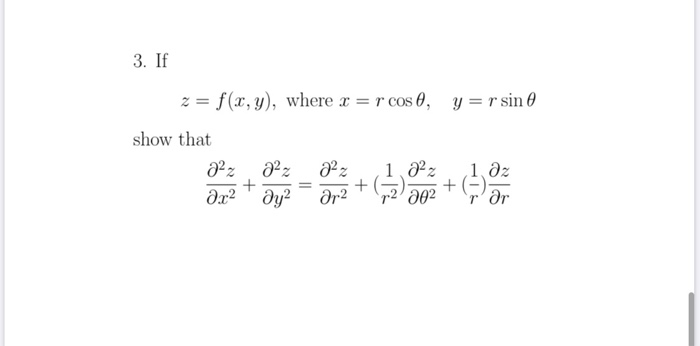3. If z = f(x,y), where x = r cos, y=r sin 0 show that 222 222 1 222 1.az + + +) ar2 ду? ar2 a02 rar

• ### (P(x),Q(y), R(z)), where P depends only 2. Let S be any surface with boundary curve C, and let F(x,y, z) on r, where Q...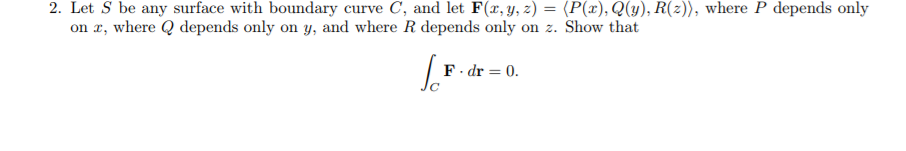(P(x),Q(y), R(z)), where P depends only 2. Let S be any surface with boundary curve C, and let F(x,y, z) on r, where Q depends only on y, and where R depends only on z. Show that F.dr 0 C (P(x),Q(y), R(z)), where P depends only 2. Let S be any surface with boundary curve C, and let F(x,y, z) on r, where Q depends only on y, and where R depends only on z. Show that F.dr 0 C

• ### 11. (8 marks) Let F(x, y, z) = x'yz, where r, y,z E R and y,...11. (8 marks) Let F(x, y, z) = x'yz, where r, y,z E R and y, z 2 0. Execute the following steps to prove that F(z,y,2) < (y 11(a) Assume each of r, y, z is non-zero and so ryz= s, where s> 0. Prove that 3 F(e.y.) (y)( su, y su, z sw and refer back to Question (Hint: Set 10.) 11(b) Show that if r 0 or y0 or z 0, then F(z, y, z) ( 11(c)...

• ### дz дz 1. In the equation, x sin y - y cos z + xyz =...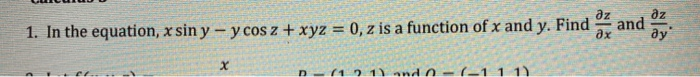дz дz 1. In the equation, x sin y - y cos z + xyz = 0, z is a function of x and y. Find and ду" дх D- 1) and o- (-11 1)

• ### A B C Parametrize, but do not evaluate, //f(x, y, z) ds, where f(x, y, z) 2y22 and S is the part , where J(,y,) 3...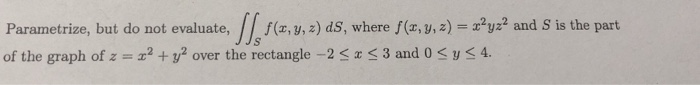A B C Parametrize, but do not evaluate, //f(x, y, z) ds, where f(x, y, z) 2y22 and S is the part , where J(,y,) 3 3 and 0 Sys4 of the graph of z2 over the rectangle -2 s . Parametrize, but do not evaluate, F.n ds, where F (,-,z) and S is the sphere of radius 2 centered at the origin. Calculate JJs xyz dS where S is the part of the cone parametrized by r(u, u) (ucos...

• ### 7. Let S = [0, 1] × [0, 1] and f : S → R be defined by f(x, y) = ( x + y, if x 2 ≤ y ≤ 2x 2 , 0, elsewhere. Show that f is integrable over S and calculate R S f(z)dz.

7. Let S = [0, 1] × [0, 1] and f : S → R be defined by f(x, y) = ( x + y, if x 2 ≤ y ≤ 2x 2 , 0, elsewhere. Show that f is integrable over S and calculate R S f(z)dz.

• ### (x, y, z) dS for the following: (a) f(x, y, z) x+4 , where S is the portion of the generalized cy...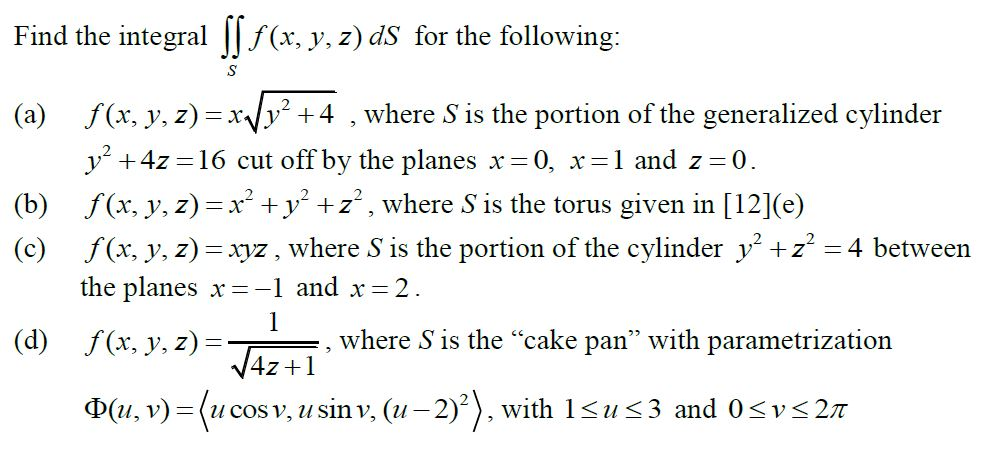Please show all work. Answers provided below Answers: (x, y, z) dS for the following: (a) f(x, y, z) x+4 , where S is the portion of the generalized cylinder y2 +4z 16 cut off by the planesx 0, x-1 and z- 0 (b) f (c) f (x, y, z)-xyz, where S is the torus given in (e) (x, y, z)-xyz, where S is the portion of the cylinder y + z the planes x-1 and x 2 f(x, y,...

• ### VIII.6.9.21. Let U be an open set in R3, and F: U R be a C...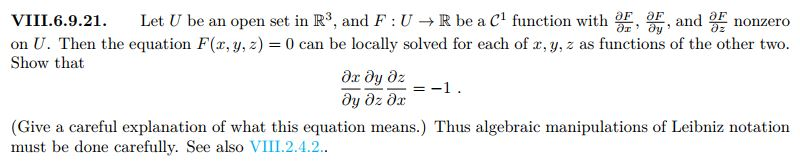VIII.6.9.21. Let U be an open set in R3, and F: U R be a C function with OFF, and nonzero on U. Then the equation F(x, y, z) = 0 can be locally solved for each of x, y, z as functions of the other two. Show that ду ду дz dy azar=-1. (Give a careful explanation of what this equation means.) Thus algebraic manipulations of Leibniz notation must be done carefully. See also VIII.2.4.2.. VIII.2.4.2. Discuss the validity...

• ### Example A.3 Surface normal vector. Let S be a surface that is represented by f(x, y, z) -c, where...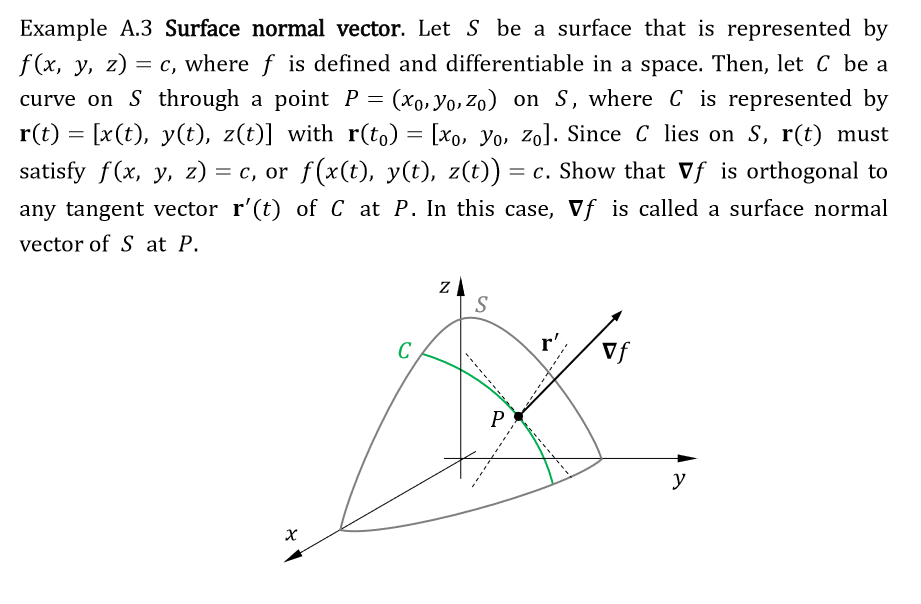Example A.3 Surface normal vector. Let S be a surface that is represented by f(x, y, z) -c, where f is defined and differentiable in a space. Then, let C be a curve on S through a point P-Go, yo,Zo) on S, where C is represented by rt)[x(t), y(t), z(t)] with r(to) -[xo. Vo, zol. Since C lies on S, r(t) must satisfy f(x, y. z)-c, or f(x(t), y(t), z(t))-c. Show that vf is orthogonal to any tangent vector r'(t)...

• ### Evaluate Z Z S curl(F) · dS where F(x, y, z) = (x^ 3 , −z...

Evaluate Z Z S curl(F) · dS where F(x, y, z) = (x^ 3 , −z ^3y ^3 , 2x − 4y) and S is the portion of the paraboloid z = x ^2 + y^ 2 − 3 below the plane z = 1 with orientation in the negative z-axis direction.